Next: Harmonic Perturbations Up: Time-Dependent Perturbation Theory Previous: Spin Magnetic Resonance

# Perturbation Expansion

Let us recall the analysis of Sect. 13.2. The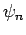are the stationary orthonormal eigenstates of the time-independent unperturbed Hamiltonian,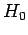. Thus,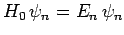, where the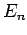are the unperturbed energy levels, and. Now, in the presence of a small time-dependent perturbation to the Hamiltonian,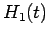, the wavefunction of the system takes the form(1057)

where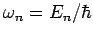. The amplitudessatisfy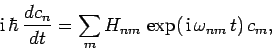(1058)

whereand. Finally, the probability of finding the system in the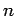th eigenstate at timeis simply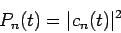(1059)

(assuing that, initially,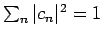).

Suppose that at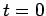the system is in some initial energy eigenstate labeled. Equation (1058) is, thus, subject to the initial condition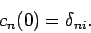(1060)

Let us attempt a perturbative solution of Eq. (1058) using the ratio ofto(or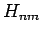to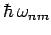, to be more exact) as our expansion parameter. Now, according to (1058), theare constant in time in the absence of the perturbation. Hence, the zeroth-order solution is simply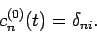(1061)

The first-order solution is obtained, via iteration, by substituting the zeroth-order solution into the right-hand side of Eq. (1058). Thus, we obtain(1062)

subject to the boundary condition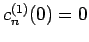. The solution to the above equation is(1063)

It follows that, up to first-order in our perturbation expansion,(1064)

Hence, the probability of finding the system in some final energy eigenstate labeledat time, given that it is definitely in a different initial energy eigenstate labeledat time, is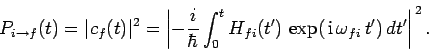(1065)

Note, finally, that our perturbative solution is clearly only valid provided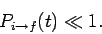(1066)Next: Harmonic Perturbations Up: Time-Dependent Perturbation Theory Previous: Spin Magnetic Resonance
Richard Fitzpatrick 2010-07-20